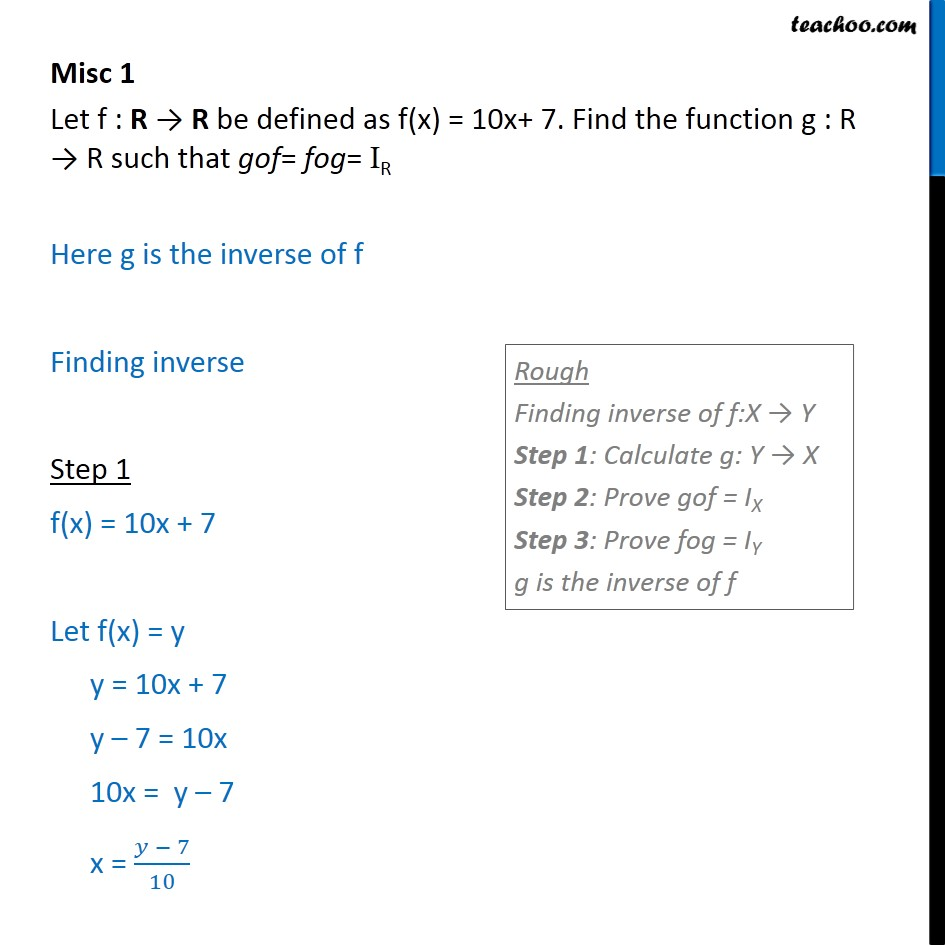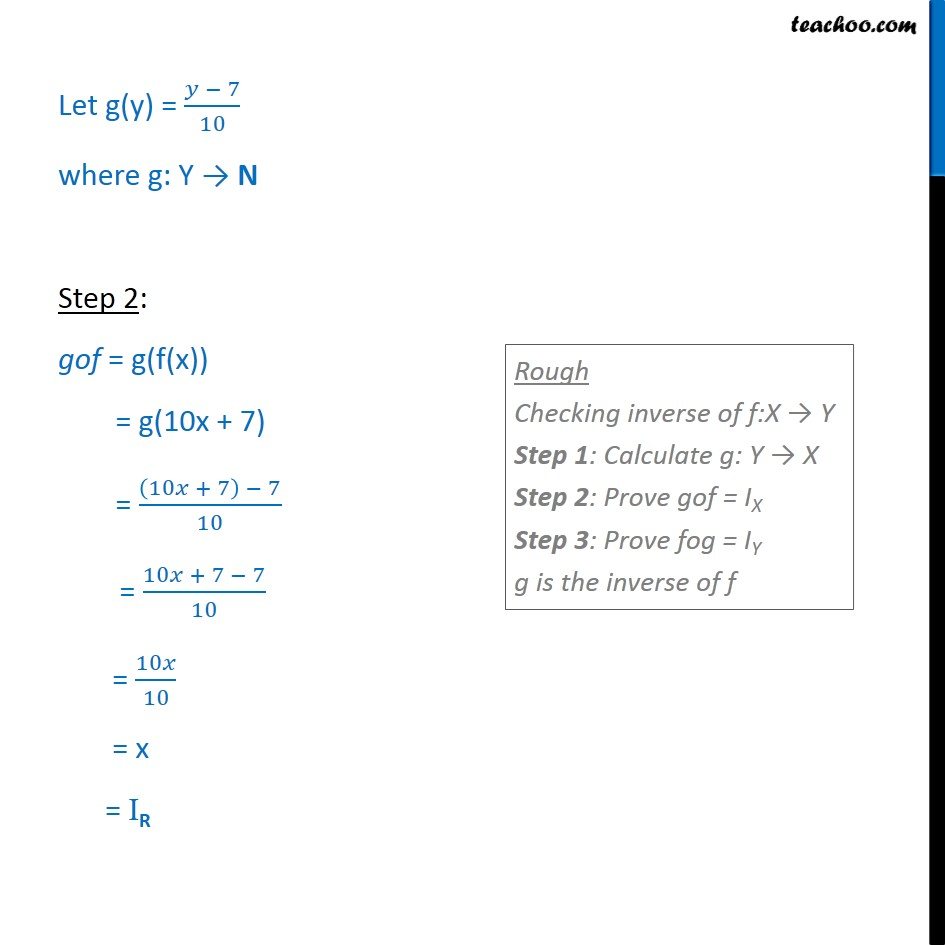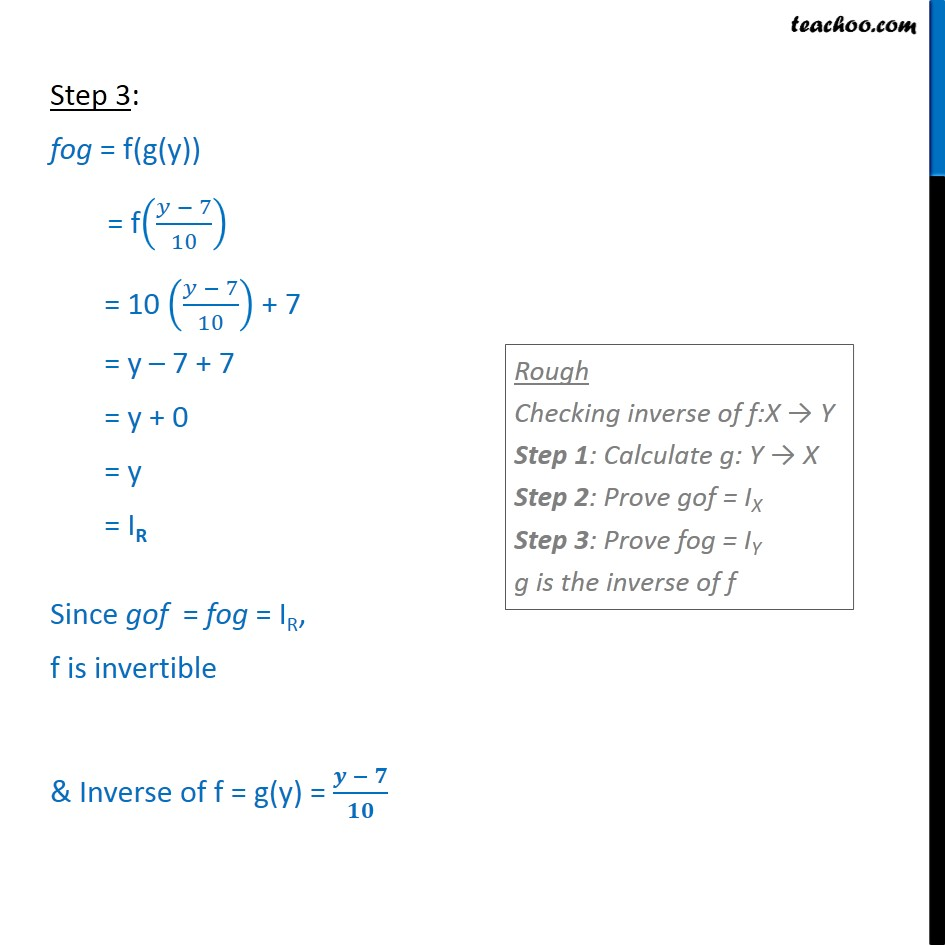1. Chapter 1 Class 12 Relation and Functions
2. Serial order wise
3. Miscellaneous

Transcript

Misc 1 Let f : R R be defined as f(x) = 10x+ 7. Find the function g : R R such that gof= fog= IR Here g is the inverse of f Finding inverse Step 1 f(x) = 10x + 7 Let f(x) = y y = 10x + 7 y 7 = 10x 10x = y 7 x = 7 10 Let g(y) = 7 10 where g: Y N Step 2: gof = g(f(x)) = g(10x + 7) = 10 + 7 7 10 = 10 + 7 7 10 = 10 10 = x = IR Step 3: fog = f(g(y)) = f((𝑦 − 7)/10) = 10 ((𝑦 − 7)/10) + 7 = y – 7 + 7 = y + 0 = y = IR Since gof = fog = IR, f is invertible & Inverse of f = g(y) = (𝒚 − 𝟕)/𝟏𝟎

Miscellaneous